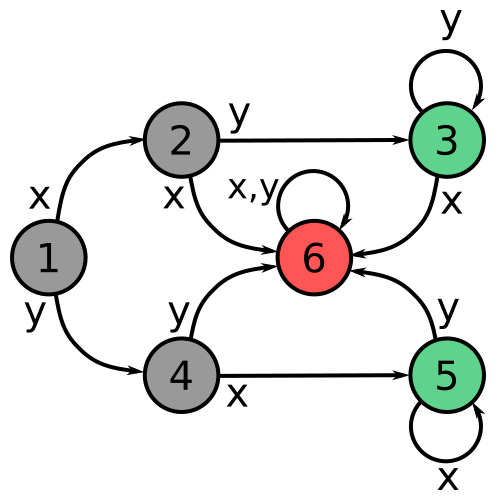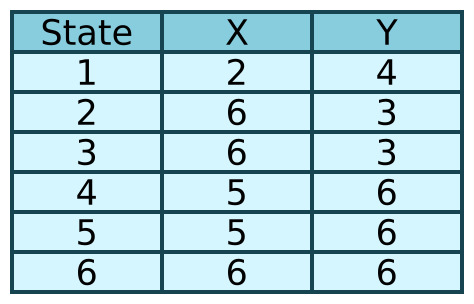# Finite state machines

Martin McBride, 2018-05-13
Tags none
Categories none

In computing we sometimes need to deal with sequences that have to happen in a certain order. Some examples are:

• a sequence of ones and zeros in a binary stream arriving over a network.
• a sequence of characters in a string. For example the string "0xAA55" correctly represents a hexadecimal number, but the string "A5xA05" does not.
• a sequence of mouse operations. For example in file explorer you click and drag a marquee tool to select a set of files, then click and drag the files to move them to another folder.

A finite state machine (FSM) is a way to model these types of sequence. It can represent all the possible states, and inputs, and what happens every case. This can be used to check if a sequence is valid according to its rules. It can also be used to extract meaning from the sequence (for example, to convert a hex string to a numerical value.)

In some cases, we actually implement a state machine in code to solve the problem. In other cases, there are better ways to implement the code, but the state machine still helps us analyse the problem.

## A simple example

Let's imagine a simple language where the only characters are X and Y. The "words" in our language consist of either an X followed by one or more Ys such as:

XY or XYY or XYYY etc

or a Y followed by one or more Xs such as

YX or YXX or YXXX etc

Here is a state transition diagram that models these sequences:## Checking a valid string

The diagram might look a bit complicated at first, but it will help to look at the valid string XYY.

1. We start in the initial state, state 1.
2. The first symbol is an X, so we take the X branch out of state 1. This leads to state 2.
3. The next symbol is a Y, so we take the Y branch out of state 2. This leads to state 3.
4. The next symbol is a Y, so we take the Y branch out of state 3. This leads back to state 3.

The string is now finished, and the state machine is left is state 3, which is one of the valid end states (marked in green), which shows that the string is valid.

Since state 3 loops back on itself when it receives a Y, then it will end up is state 3 for all input strings of form XY, XYY, XYYY etc for any number of trailing Ys.

If you work through the same process for input string YXX, you should see that it passes through state 4 to state 5, which is also a valid end state.

## Checking an invalid string

Let's look at some invalid cases.

First, the string XX. Here is what happens:

1. We start in the initial state, state 1.
2. The first symbol is an X, so we take the X branch out of state 1. This leads to state 2.
3. The next symbol is an X, so we take the X branch out of state 2. This leads to state 6.

State 6 is an error state. If we arrive there, it is because the string is invalid.

Notice that, once in state 6 there is no escape. If the string had more characters, for example XXYX, those extra characters would just lead back to state 6.

Here is another error case, the string XYX. Here is what happens this time

1. We start in the initial state, state 1.
2. The first symbol is an X, so we take the X branch out of state 1. This leads to state 2.
3. The next symbol is a Y, so we take the Y branch out of state 2. This leads to state 3.
4. The next symbol is an X, so we take the X branch out of state 3. This leads to state 6.

So we arrive at the error state again, because once again it is in an invalid string.

Finally, consider the string X:

1. We start in the initial state, state 1.
2. The first symbol is an X, so we take the X branch out of state 1. This leads to state 2.

Now the string has ended, and we are still in state 2. This isn't an error state, but it isn't a valid end state either. This tells us that the string isn't valid (but it is because itis incomplete rather than having an actual error).

Of course, similar errors occur for strings YY, YXY and Y.

## Representing the state machine as a table

We can represent the state machine as a state transition table, like this:The first column represents the current state. The X column represents the next state if the next input is an X. The Y column represents the next state if the next input is a Y. The table contains exactly the same information as the diagram, just in a different form.

In the next section we will look at how a state machine can be used to convert hexadecimal, and how we might implement a state machine in code.